## Overview: OPTLP Procedure

The OPTLP procedure provides four methods of solving linear programs (LPs). A linear program has the following formulation: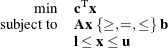where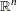is the vector of decision variables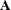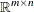is the matrix of constraintsis the vector of objective function coefficients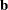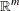is the vector of constraints right-hand sides (RHS)is the vector of lower bounds on variablesis the vector of upper bounds on variables

The following LP solvers are available in the OPTLP procedure:

• primal simplex solver

• dual simplex solver

• network simplex solver

• interior point solver

The primal and dual simplex solvers implement the two-phase simplex method. In phase I, the solver tries to find a feasible solution. If no feasible solution is found, the LP is infeasible; otherwise, the solver enters phase II to solve the original LP. The network simplex solver extracts a network substructure, solves this using network simplex, and then constructs an advanced basis to feed to either primal or dual simplex. The interior point solver implements a primal-dual predictor-corrector interior point algorithm.

PROC OPTLP requires a linear program to be specified using a SAS data set that adheres to the MPS format, a widely accepted format in the optimization community. For details about the MPS format see Chapter 15: The MPS-Format SAS Data Set.

You can use the MPSOUT= option to convert typical PROC LP format data sets into MPS-format SAS data sets. The option is available in the LP, INTPOINT, and NETFLOW procedures. For details about this option, see Chapter 5: The LP Procedure in SAS/OR 12.3 User's Guide: Mathematical Programming Legacy Procedures, Chapter 4: The INTPOINT Procedure in SAS/OR 12.3 User's Guide: Mathematical Programming Legacy Procedures, and Chapter 6: The NETFLOW Procedure in SAS/OR 12.3 User's Guide: Mathematical Programming Legacy Procedures.